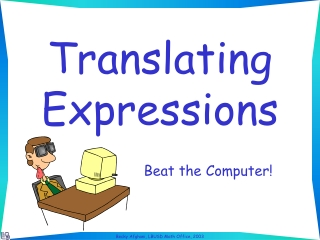DownloadDownload PresentationTranslating Expressions

# Translating Expressions

Download Presentation## Translating Expressions

- - - - - - - - - - - - - - - - - - - - - - - - - - - E N D - - - - - - - - - - - - - - - - - - - - - - - - - - -
##### Presentation Transcript

1. Translating Expressions Beat the Computer!

2. Directions: • Say the expression out loud before the computer gives you the answer • Use n for the variable • The slides will advance automatically

3. The difference of eleven and a number

4. Twenty less than a number

5. The sum of six and a number

6. Sixteen less than a number

7. The quotient of a number and six

8. Three less than a number

9. A number increased by seventeen

10. The product of twelve and a number

11. Eight subtracted from a number

12. A number take away sixty

13. Twice a number

14. A number multiplied by four, less five

15. Seven divided by a number, plus four

16. Five fewer than a number

17. A number decreased by forty

18. Double a number, less eleven

19. Double a number, less eleven 2n - 11

20. Six more than a number, all divided by three